﻿ 基于AMESim水下复杂均衡系统仿真及优化
 舰船科学技术2022, Vol. 44Issue (19): 38-41    DOI: 10.3404/j.issn.1672-7649.2022.19.008PDF

Simulation and optimization of underwater complex equalization system based on AMESim
QIAN Yu, XU Ren-chao, HU Hao-long
Taihu Laboratory of Deep-Sea Technological Science, China Ship Scientific Research Center, Wuxi, 214082, China
Abstract: Aiming at the problem that the submersible buoyancy adjustment system and the trim balancing system are independent of each other and occupy a large space, this paper proposed a complex underwater balancing system. However, in the balancing process of the system, due to the sealing of the front and rear water tanks, it is easy to cause unequal water tank loads, resulting in a large difference in the flow of the two water tanks in the process of water injection and drainage. Therefore, the optimal design of the flow divider and combiner valve is adopted to solve the problem of uneven flow distribution in the process of water injection and drainage. Through the establishment of AMESim simulation models respectively, the flow characteristics of the water injection and drainage process of the system are studied and analyzed. The simulation results show that after the use of the flow divider and combiner valve in this complex equalization system, the distribution of the injection and drainage flow is obviously more even, with the single diverting accuracy as high as 3.8% and the single collecting accuracy as high as 2.4%.
Key words: balancing system     flow divider and combiner valve     flow distribution     AMESim
0 引　言

1 均衡系统设计 1.1 工作原理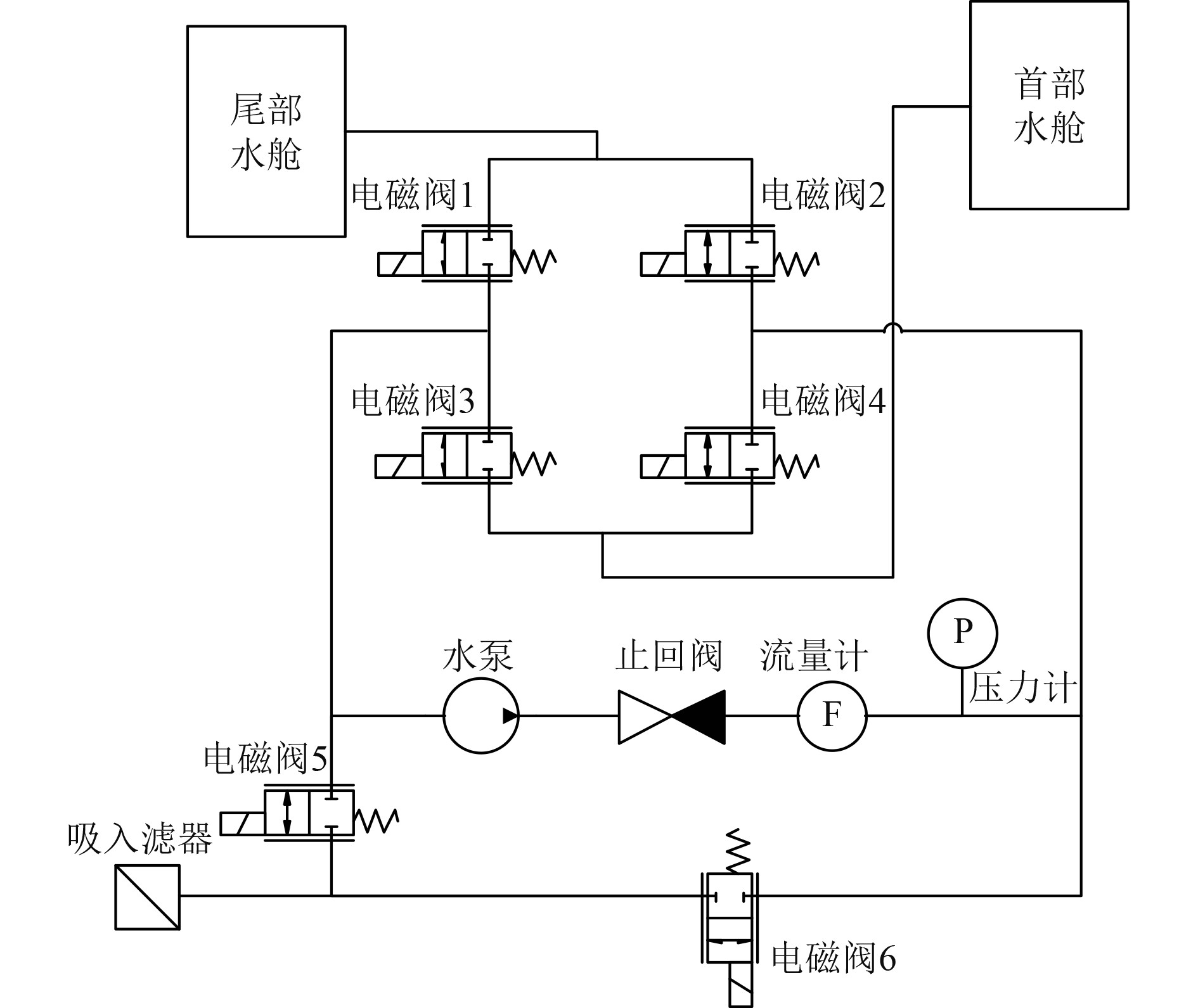图 1 均衡系统原理图 Fig. 1 Schematic diagram of equalization system

1.2 分流集流阀流量方程

 ${Q_1} = {C_d} \cdot \frac{{{\text{π}} d_1^2}}{4}\sqrt {\frac{{2({P_0} - {P_1})}}{\rho }}，$ (1)
 ${Q_2} = {C_d} \cdot \frac{{{\text{π}}d_2^2}}{4}\sqrt {\frac{{2({P_0} - {P_2})}}{\rho }} 。$ (2)

 ${Q_A} = {C_d} \cdot {\text{π}} \cdot {W_1}x\sqrt {\frac{{2({P_1} - {P_A})}}{\rho }} ，$ (3)
 ${Q_B} = {C_d} \cdot {\text{π}} \cdot {W_2}x\sqrt {\frac{{2({P_2} - {P_B})}}{\rho }} ，$ (4)

 ${P_{a1}}{V_{a1}} = {P_{a2}}{V_{a2}} ，$ (5)
 ${V_{a2}} = V - {V_{a1}} - qt 。$ (6)

 $\delta = \frac{{\left| {{Q_A} - {Q_B}} \right|}}{{{Q_A} + {Q_B}}} \times 100{\text{%}} 。$ (7)

1.3 优化后的均衡系统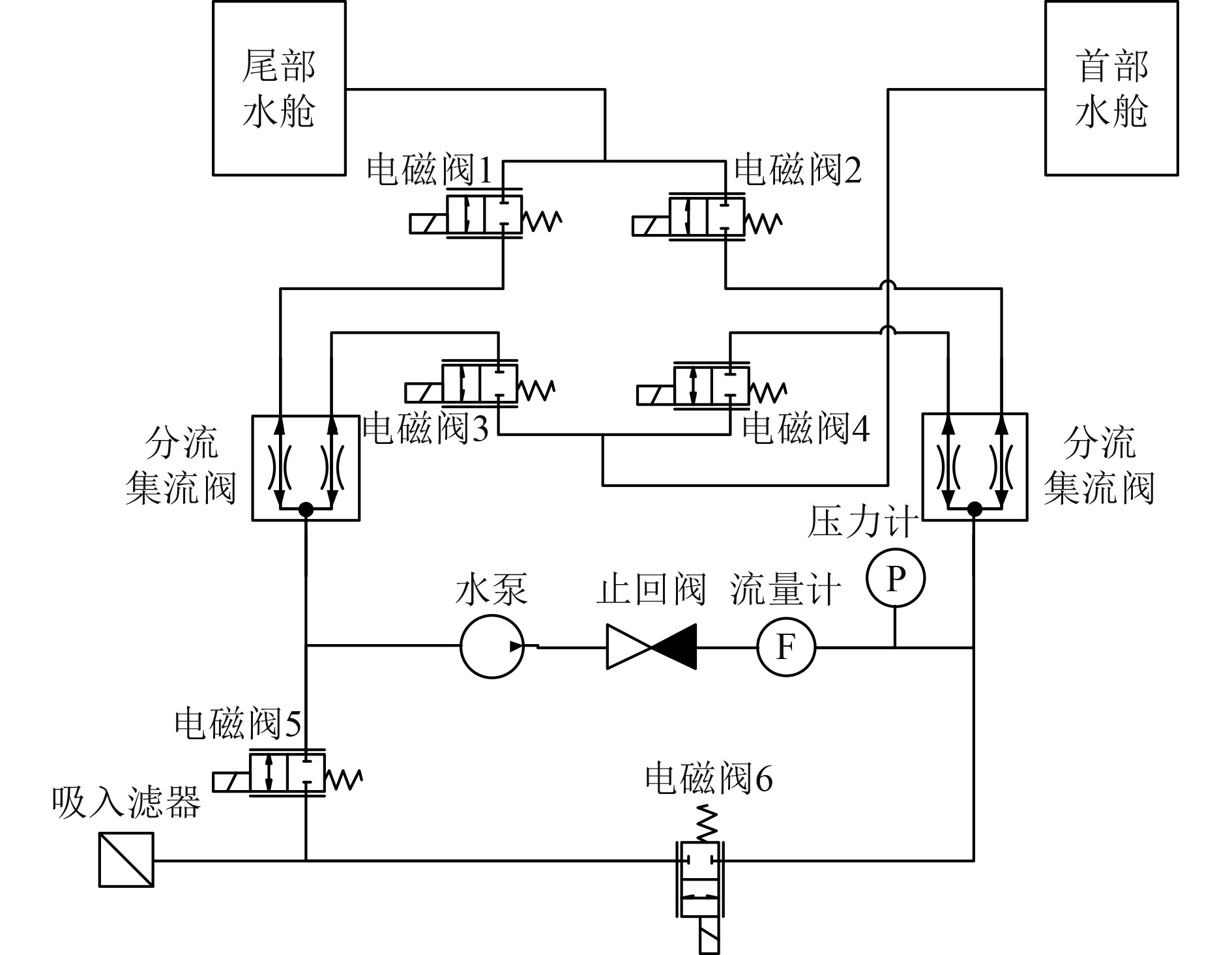图 2 优化后的均衡系统 Fig. 2 Optimized equalization system
2 仿真模型的建立 2.1 分流集流阀模型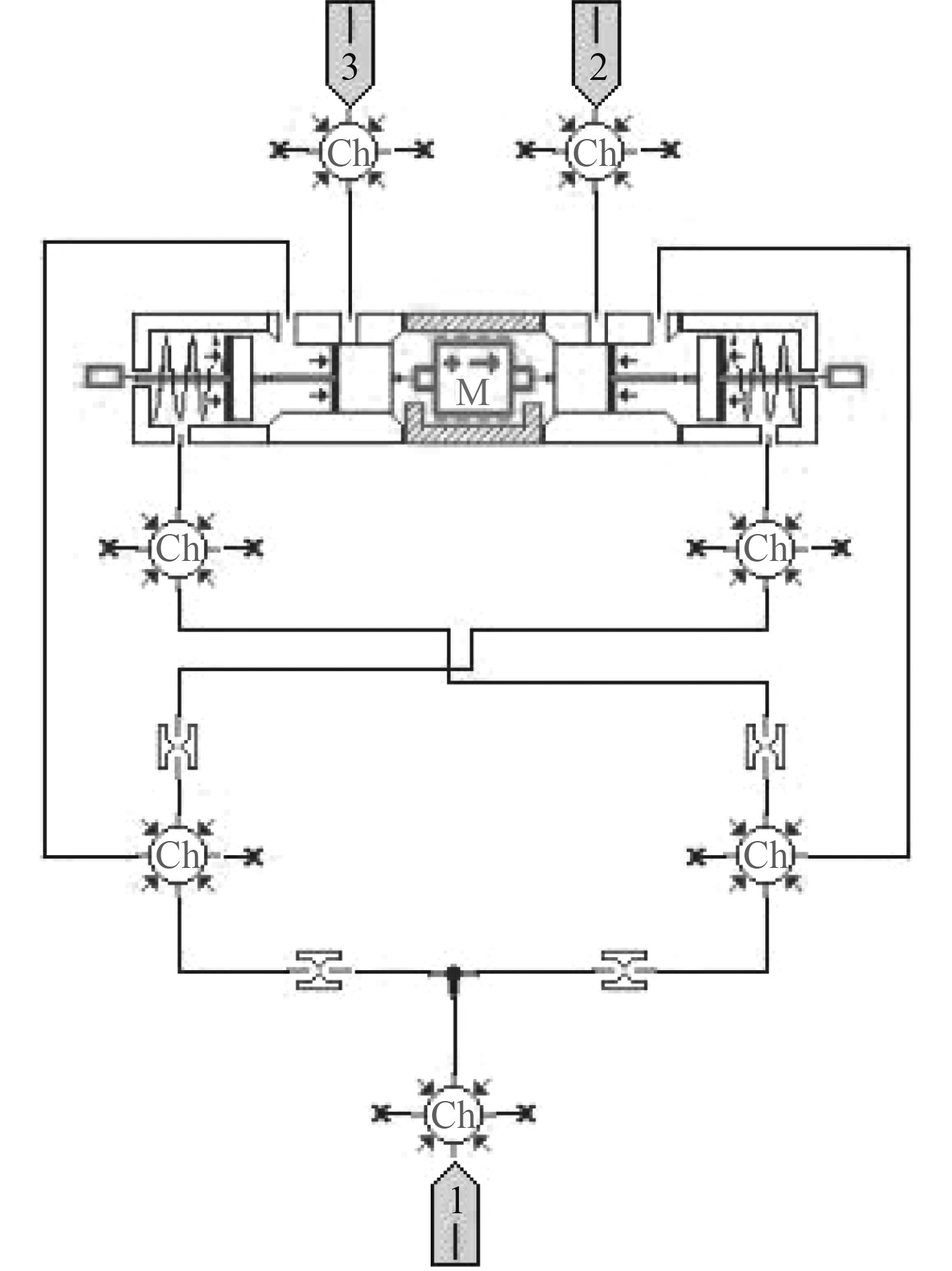图 3 分流集流阀模型 Fig. 3 Model of flow divider and combiner valve表 1 分流集流阀模型参数 Tab.1 The parameter of flow divider and combiner valve
2.2 均衡系统模型

3 仿真结果分析 3.1 参数设置表 2 均衡系统工况顺序表 Tab.2 The operating sequence table of equalization system
3.2 仿真结果分析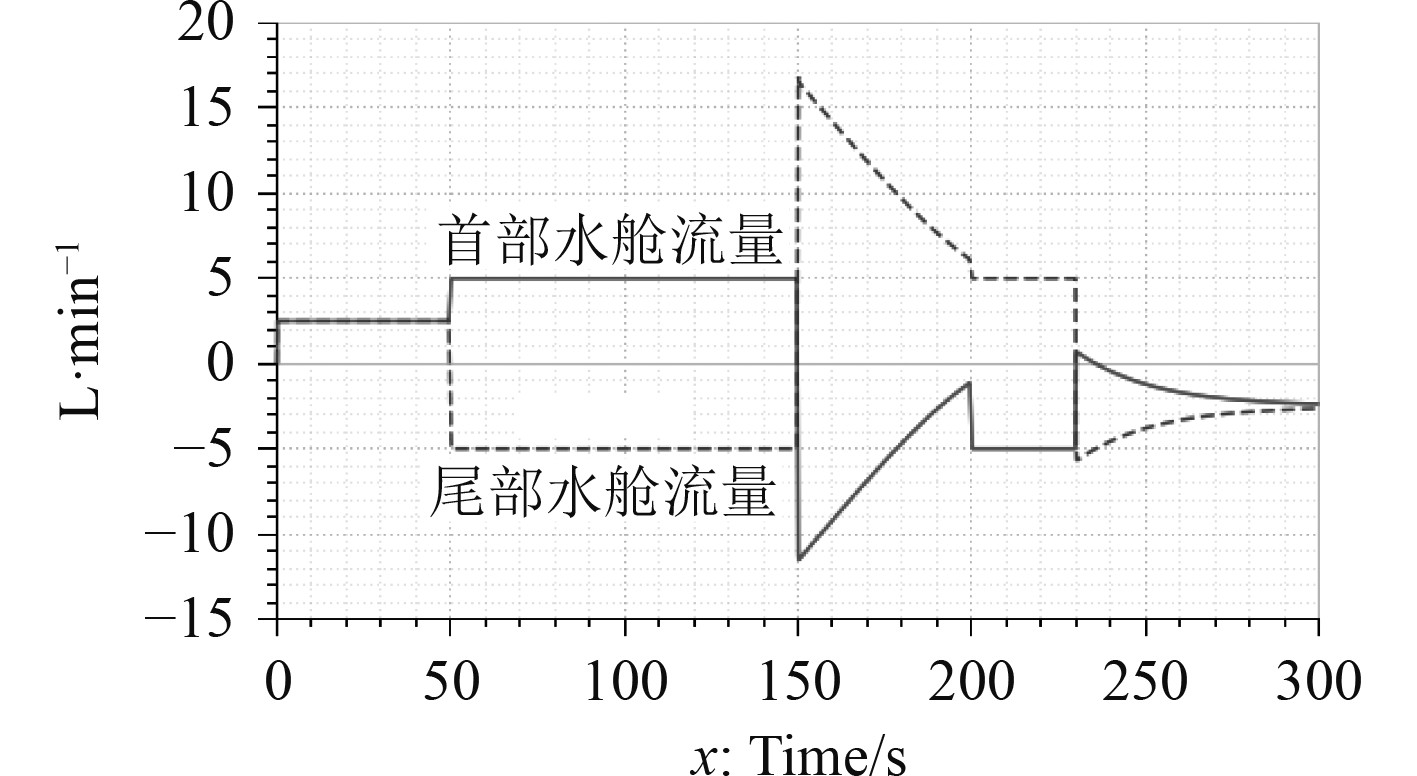图 4 优化前均衡系统流量曲线图 Fig. 4 Flow chart of equalization system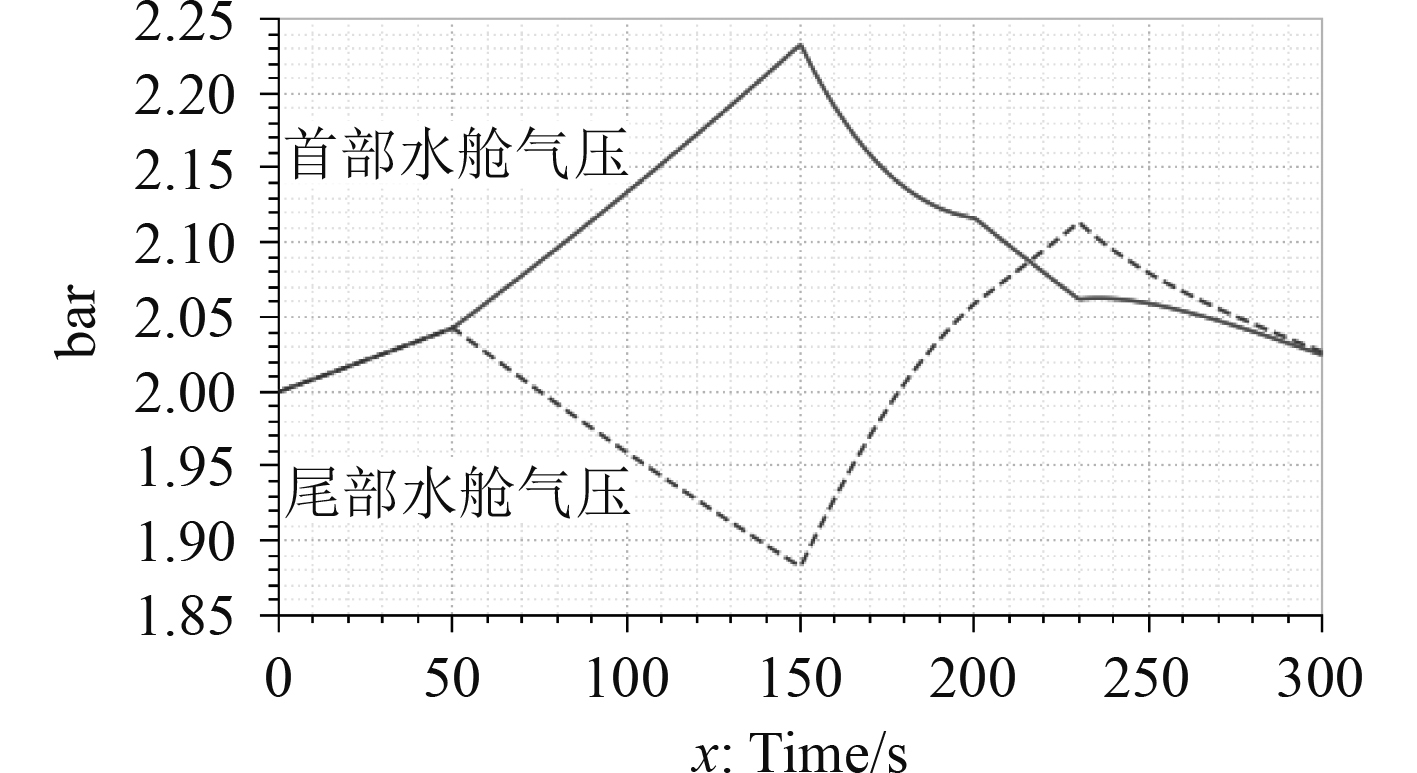图 5 首尾水舱空气压力曲线图 Fig. 5 Air pressure curve of water tanks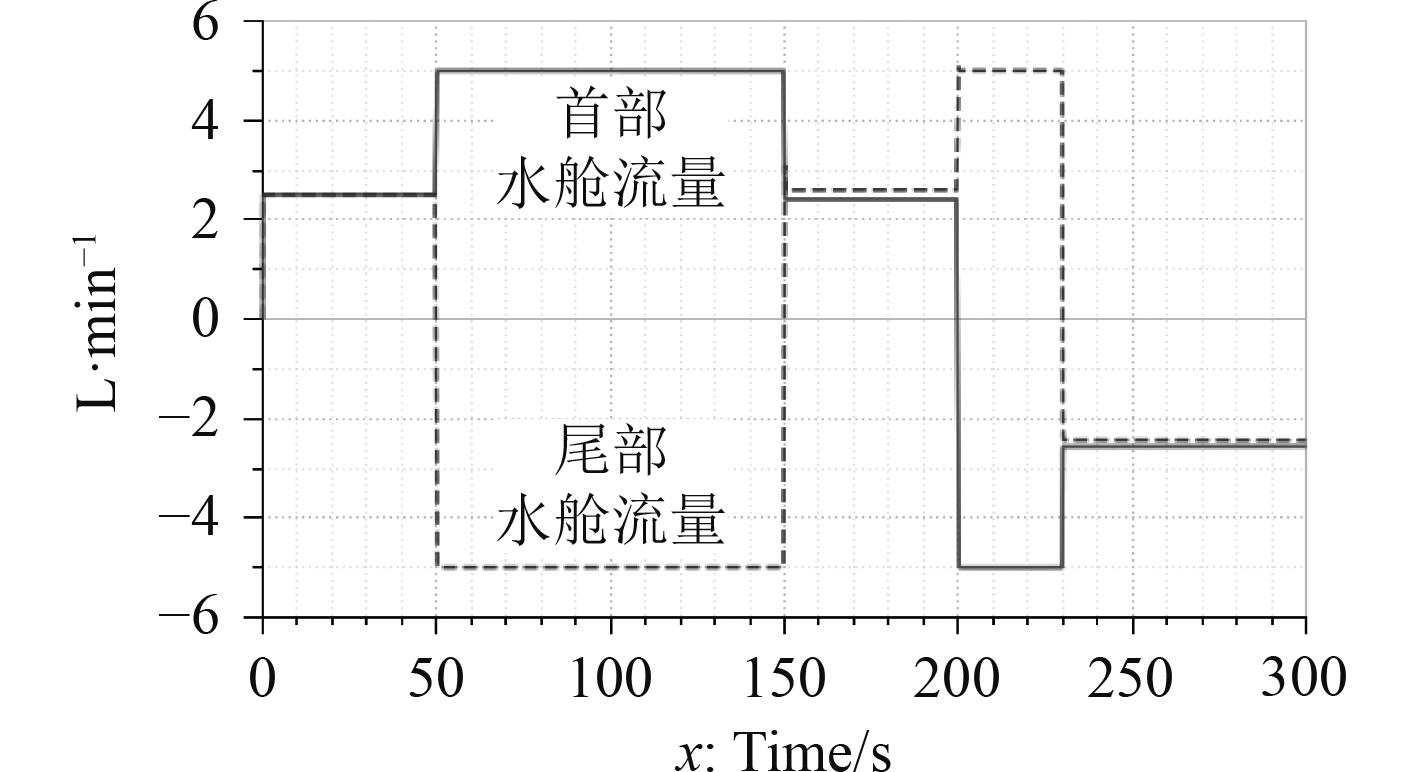图 6 优化后均衡系统流量曲线 Fig. 6 Flow chart of optimized equalization system图 7 优化后水舱气压曲线图 Fig. 7 Air pressure curve of water tanks

4 结　语

1）本文提出一种复杂均衡系统的原理方案，该系统采用单一泵源和阀组，实现了浮力调节和纵倾平衡的功能。通过理论分析，发现注排水流量不均的问题。因此，提出基于分流集流阀的优化方案。

2）分别建立优化前后的均衡系统AMESim系统模型，通过同一工况仿真对比。结果表明，明显改善了不同密闭水舱注排水流量不均的问题，单路流量分流精度达3.8%，集流精度达2.4%。

  张希贤, 唐荣庆. 潜艇装置及系统[M]. 上海: 上海交通大学出版社, 1996.  邱中梁, 王璇. 液压油泵送水银式纵倾调节系统设计研究[J]. 液压气动与密封, 2007, 27(5): 2. QIU Z L, WANG X. Design research about trim system with hydraulic oil pumping mercury[J]. Hydraulics Pneumatics & Seals, 2007, 27(5): 2. DOI:10.3969/j.issn.1008-0813.2007.05.009  刘银水, 吴德发, 李东林, 等. 大深度潜水器海水液压浮力调节技术研究进展[J]. 液压与气动, 2014(10): 10. LIU Y S, WU D F, LI D L, et al. Seawater hydraulic buoyancy adjusting system for large-depth submersible[J]. Chinese Hydraulics & Pneumatics, 2014(10): 10. DOI:10.11832/j.issn.1000-4858.2014.10.001  高世阳, 崔汉国, 张奇峰, 等. 深海油囊式浮力调节系统的研制[J]. 液压与气动, 2016(10): 75-80. GAO S Y, CUI H G, ZHANG Q F, et al. The development of a deep-sea oil bladder type buoyancy adjustment system[J]. Chinese Hydraulics & Pneumatics, 2016(10): 75-80. DOI:10.11832/j.issn.1000-4858.2016.010.014  尹远, 刘铁军, 徐会希, 等. AUV用高精度吸排油浮力调节系统[J]. 海洋技术学报, 2018, 37(5): 6.  金荣, 黄政, 胡华兵, 等. 潜艇均衡系统自流注水调节阀稳态噪声试验研究[J]. 舰船科学技术, 2021, 43(7): 98-101. JIN R, HUANG Z, HU H B, etc. Test study on steady noise of the self flowing water injection flow control valve in submarine balanced system[J]. Ship Science and Technology, 2021, 43(7): 98-101. DOI:10.3404/j.issn.1672-7649.2021.04.020  孟路, 仲晨华. 潜艇悬停水舱排注水控制方式比较[J]. 船海工程, 2014, 43(4): 160-162. MENG L, ZHONG C H. Comparison of the control mode of water injection and drainage for the submarine hovering water tank[J]. Ship & Ocean Engineering, 2014, 43(4): 160-162. DOI:10.3963/j.issn.1671-7953.2014.04.041